# Test: Flow Through Pipes - 2

## 10 Questions MCQ Test Topicwise Question Bank for Civil Engineering | Test: Flow Through Pipes - 2

Description
Attempt Test: Flow Through Pipes - 2 | 10 questions in 30 minutes | Mock test for Civil Engineering (CE) preparation | Free important questions MCQ to study Topicwise Question Bank for Civil Engineering for Civil Engineering (CE) Exam | Download free PDF with solutions
QUESTION: 1

### Two reservoirs at different levels are connected by two parallel pipes of diameter '2d' and 'd'. The ratio of the flows in the two pipes (larger: smaller) is

Solution: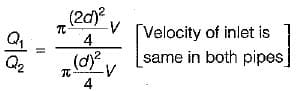⇒ Q1/Q2 = 4/1

QUESTION: 2

### A 15 cm diameter pipe carries a flow of 70 L/s of an oil (RD = 0.75). At a section 12 cm above the datum the pressure is vacuum of 2 cm of mercury, if the kinetic energy correction factor a for this section is 1.1, the total head at the section in metres of oil is

Solution:

Total head =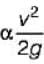+ ρ/y + z (here p is negative).

QUESTION: 3

### The loss of head due to friction in a pipe of uniform diameter in which viscous flow is taking place is where Re = flow Reynold’s number

Solution:
QUESTION: 4

In the Moody diagram the third parameter is ks/D. Here ks is

Solution:
QUESTION: 5

A compound pipe of diameters d1, d2 and d3 having length l1, l2 and l3 respectively, is to be replaced by an equivalent pipe of uniform diameter d and length l. The size of the equivalent pipe is given by

Solution:

For pipes in series equivalent pipe is given by: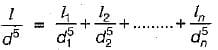For pipes in parallel equivalent pipe is given by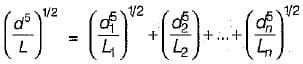QUESTION: 6

In a pipe flow, the shear velocity u. is related to the Darcy-Weisbach friction factor f as u./V =

Solution:

friction factor,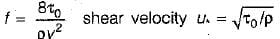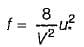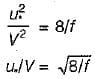QUESTION: 7

Two pipe systems are said to be equivalent when

Solution:
QUESTION: 8

An oil of kinematic viscosity 0.25 stokes flows, through a pipe of diameter 10 cm. The flow is critical., at a velocity of

Solution:

R= 2000 = VD/v
2000 =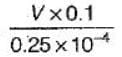⇒ V = 0.5 m/sec

QUESTION: 9

Which one of the following phenomena in a pipe flow is termed as water hammer?

Solution:
QUESTION: 10

The pressure at the summit of a syphon is

Solution:

The centre line of pipe is well above the hydraulic grade line and therefore the pressure is negative or below atmospheric.Use Code STAYHOME200 and get INR 200 additional OFF Use Coupon Code